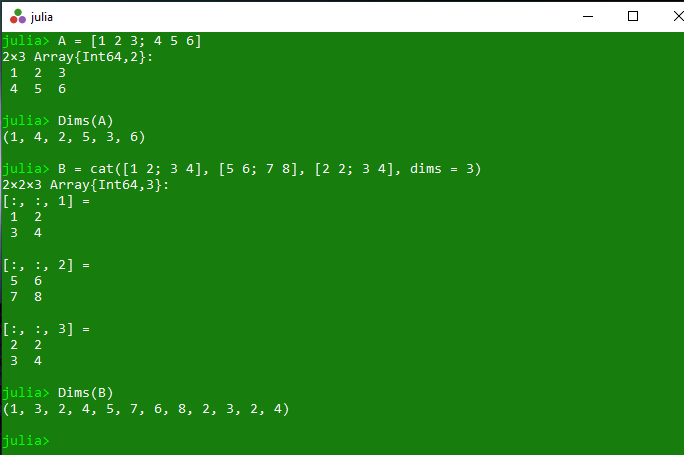Related Articles

# Representing the dimensions of array in Julia – Dims() Method

• Last Updated : 21 Apr, 2020

The `Dims()` is an inbuilt function in julia which is used to represent the dimensions of the specified AbstractArray.

Syntax: Dims(A)

Parameters:

• A: Specified array

Returns: It returns the represented dimensions of the specified AbstractArray.

Example 1:

 `# Julia program to illustrate ``# the use of Dims() method`` ` `# Getting the represented dimensions``# of the specified AbstractArray.``A ``=` `[``1``, ``2``, ``3``, ``4``]``println(Dims(A))`` ` `B ``=` `(``5``, ``10``, ``15``, ``20``)``println(Dims(B))`

Output:

```(1, 2, 3, 4)
(5, 10, 15, 20)
```

Example 2:

 `# Julia program to illustrate ``# the use of Dims() method`` ` `# Getting the represented dimensions``# of the specified AbstractArray.``A ``=` `[``1` `2` `3``; ``4` `5` `6``]``println(Dims(A))`` ` `B ``=` `cat([``1` `2``; ``3` `4``], [``5` `6``; ``7` `8``], [``2` `2``; ``3` `4``], dims ``=` `3``)``println(Dims(B))`

Output:My Personal Notes arrow_drop_up